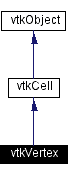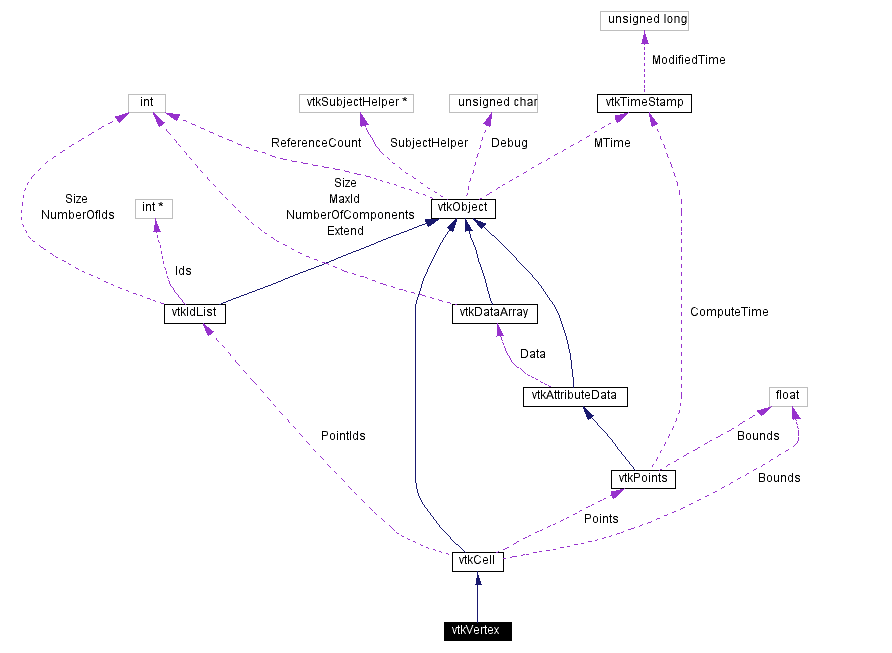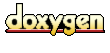Main Page   Class Hierarchy   Alphabetical List   Compound List   File List   Compound Members   File Members   Related Pages

# vtkVertex Class Reference

a cell that represents a 3D point. More...

`#include <vtkVertex.h>`

Inheritance diagram for vtkVertex:[legend]
Collaboration diagram for vtkVertex:[legend]
List of all members.

## Public Methods

virtual const char * GetClassName ()
virtual int IsA (const char *type)
vtkCellMakeObject ()
int GetCellType ()
int GetCellDimension ()
int GetNumberOfEdges ()
int GetNumberOfFaces ()
vtkCellGetEdge (int)
vtkCellGetFace (int)
void Clip (float value, vtkScalars *cellScalars, vtkPointLocator *locator, vtkCellArray *pts, vtkPointData *inPd, vtkPointData *outPd, vtkCellData *inCd, int cellId, vtkCellData *outCd, int insideOut)
int EvaluatePosition (float x, float *closestPoint, int &subId, float pcoords, float &dist2, float *weights)
void EvaluateLocation (int &subId, float pcoords, float x, float *weights)
int CellBoundary (int subId, float pcoords, vtkIdList *pts)
void Contour (float value, vtkScalars *cellScalars, vtkPointLocator *locator, vtkCellArray *verts1, vtkCellArray *lines, vtkCellArray *verts2, vtkPointData *inPd, vtkPointData *outPd, vtkCellData *inCd, int cellId, vtkCellData *outCd)
int IntersectWithLine (float p1, float p2, float tol, float &t, float x, float pcoords, int &subId)
int Triangulate (int index, vtkIdList *ptIds, vtkPoints *pts)
void Derivatives (int subId, float pcoords, float *values, int dim, float *derivs)
int CellBoundary (int subId, float pcoords, vtkIdList &pts)
int Triangulate (int index, vtkIdList &ptIds, vtkPoints &pts)

## Static Public Methods

vtkVertex * New ()
int IsTypeOf (const char *type)
vtkVertex * SafeDownCast (vtkObject *o)
void InterpolationFunctions (float pcoords, float weights)

## Protected Methods

vtkVertex ()
~vtkVertex ()
vtkVertex (const vtkVertex &)
void operator= (const vtkVertex &)

## Detailed Description

a cell that represents a 3D point.

Date:
2000/12/10 20:08:21
Revision:
1.50

vtkVertex is a concrete implementation of vtkCell to represent a 3D point.

Examples:
vtkVertex (examples)

Definition at line 57 of file vtkVertex.h.

## Constructor & Destructor Documentation

 vtkVertex::vtkVertex ( ) ` [protected]`

 vtkVertex::~vtkVertex ( ) ` [inline, protected]`
 Definition at line 128 of file vtkVertex.h.

 vtkVertex::vtkVertex ( const vtkVertex & ) ` [inline, protected]`
 Definition at line 129 of file vtkVertex.h.

## Member Function Documentation

 vtkVertex* vtkVertex::New ( ) ` [static]`
 Create an object with Debug turned off, modified time initialized to zero, and reference counting on. Reimplemented from vtkObject.

 virtual const char* vtkVertex::GetClassName ( ) ` [virtual]`
 Return the class name as a string. This method is defined in all subclasses of vtkObject with the vtkTypeMacro found in vtkSetGet.h. Reimplemented from vtkCell.

 int vtkVertex::IsTypeOf ( const char * type ) ` [static]`
 Return 1 if this class type is the same type of (or a subclass of) the named class. Returns 0 otherwise. This method works in combination with vtkTypeMacro found in vtkSetGet.h. Reimplemented from vtkCell.

 virtual int vtkVertex::IsA ( const char * type ) ` [virtual]`
 Return 1 if this class is the same type of (or a subclass of) the named class. Returns 0 otherwise. This method works in combination with vtkTypeMacro found in vtkSetGet.h. Reimplemented from vtkCell.

 vtkVertex* vtkVertex::SafeDownCast ( vtkObject * o ) ` [static]`
 Will cast the supplied object to vtkObject* is this is a safe operation (i.e., a safe downcast); otherwise NULL is returned. This method is defined in all subclasses of vtkObject with the vtkTypeMacro found in vtkSetGet.h. Reimplemented from vtkCell.

 vtkCell* vtkVertex::MakeObject ( ) ` [virtual]`
 Make a new vtkVertex object with the same information as this object. Reimplemented from vtkCell.

 int vtkVertex::GetCellType ( ) ` [inline, virtual]`
 See the vtkCell API for descriptions of these methods. Reimplemented from vtkCell. Definition at line 67 of file vtkVertex.h.

 int vtkVertex::GetCellDimension ( ) ` [inline, virtual]`
 Return the topological dimensional of the cell (0,1,2, or 3). Reimplemented from vtkCell. Definition at line 68 of file vtkVertex.h.

 int vtkVertex::GetNumberOfEdges ( ) ` [inline, virtual]`
 Return the number of edges in the cell. Reimplemented from vtkCell. Definition at line 69 of file vtkVertex.h.

 int vtkVertex::GetNumberOfFaces ( ) ` [inline, virtual]`
 Return the number of faces in the cell. Reimplemented from vtkCell. Definition at line 70 of file vtkVertex.h.

 vtkCell* vtkVertex::GetEdge ( int edgeId ) ` [inline, virtual]`
 Return the edge cell from the edgeId of the cell. Reimplemented from vtkCell. Definition at line 71 of file vtkVertex.h.

 vtkCell* vtkVertex::GetFace ( int faceId ) ` [inline, virtual]`
 Return the face cell from the faceId of the cell. Reimplemented from vtkCell. Definition at line 72 of file vtkVertex.h.

 void vtkVertex::Clip ( float value, vtkScalars * cellScalars, vtkPointLocator * locator, vtkCellArray * pts, vtkPointData * inPd, vtkPointData * outPd, vtkCellData * inCd, int cellId, vtkCellData * outCd, int insideOut ) ` [virtual]`
 Cut (or clip) the cell based on the input cellScalars and the specified value. The output of the clip operation will be one or more cells of the same topological dimension as the original cell. The flag insideOut controls what part of the cell is considered inside - normally cell points whose scalar value is greater than "value" are considered inside. If insideOut is on, this is reversed. Also, if the output cell data is non-NULL, the cell data from the clipped cell is passed to the generated contouring primitives. (Note: the CopyAllocate() method must be invoked on both the output cell and point data. The cellId refers to the cell from which the cell data is copied.) Reimplemented from vtkCell.

 int vtkVertex::EvaluatePosition ( float x, float * closestPoint, int & subId, float pcoords, float & dist2, float * weights ) ` [virtual]`
 Given a point x return inside(=1) or outside(=0) cell; evaluate parametric coordinates, sub-cell id (!=0 only if cell is composite), distance squared of point x to cell (in particular, the sub-cell indicated), closest point on cell to x (unless closestPoint is null, in which case, the closest point and dist2 are not found), and interpolation weights in cell. (The number of weights is equal to the number of points defining the cell). Note: on rare occasions a -1 is returned from the method. This means that numerical error has occurred and all data returned from this method should be ignored. Also, inside/outside is determine parametrically. That is, a point is inside if it satisfies parametric limits. This can cause problems for cells of topological dimension 2 or less, since a point in 3D can project onto the cell within parametric limits but be "far" from the cell. Thus the value dist2 may be checked to determine true in/out. Reimplemented from vtkCell.

 void vtkVertex::EvaluateLocation ( int & subId, float pcoords, float x, float * weights ) ` [virtual]`
 Determine global coordinate (x) from subId and parametric coordinates. Also returns interpolation weights. (The number of weights is equal to the number of points in the cell.) Reimplemented from vtkCell.

 int vtkVertex::CellBoundary ( int subId, float pcoords, vtkIdList * pts ) ` [virtual]`
 Given parametric coordinates of a point, return the closest cell boundary, and whether the point is inside or outside of the cell. The cell boundary is defined by a list of points (pts) that specify a vertex (1D cell). If the return value of the method is != 0, then the point is inside the cell. Reimplemented from vtkCell.

 void vtkVertex::Contour ( float value, vtkScalars * cellScalars, vtkPointLocator * locator, vtkCellArray * verts1, vtkCellArray * lines, vtkCellArray * verts2, vtkPointData * inPd, vtkPointData * outPd, vtkCellData * inCd, int cellId, vtkCellData * outCd ) ` [virtual]`
 Generate contouring primitives. The scalar list cellScalars are scalar values at each cell point. The point locator is essentially a points list that merges points as they are inserted (i.e., prevents duplicates). Reimplemented from vtkCell.

 int vtkVertex::IntersectWithLine ( float p1, float p2, float tol, float & t, float x, float pcoords, int & subId ) ` [virtual]`
 Intersect with a ray. Return parametric coordinates (both line and cell) and global intersection coordinates, given ray definition and tolerance. The method returns non-zero value if intersection occurs. Reimplemented from vtkCell.

 int vtkVertex::Triangulate ( int index, vtkIdList * ptIds, vtkPoints * pts ) ` [virtual]`
 Triangulate the vertex. This method fills pts and ptIds with information from the only point in the vertex. Reimplemented from vtkCell.

 void vtkVertex::Derivatives ( int subId, float pcoords, float * values, int dim, float * derivs ) ` [virtual]`
 Get the derivative of the vertex. Returns (0.0, 0.0, 0.0) for all dimensions. Reimplemented from vtkCell.

 void vtkVertex::InterpolationFunctions ( float pcoords, float weights ) ` [static]`
 Vertex specific methods.

 int vtkVertex::CellBoundary ( int subId, float pcoords, vtkIdList & pts ) ` [inline]`
 For legacy compatibility. Do not use. Definition at line 120 of file vtkVertex.h.

 int vtkVertex::Triangulate ( int index, vtkIdList & ptIds, vtkPoints & pts ) ` [inline]`
 Definition at line 122 of file vtkVertex.h.

 void vtkVertex::operator= ( const vtkVertex & ) ` [inline, protected]`
 Definition at line 130 of file vtkVertex.h.

The documentation for this class was generated from the following file:

Generated on Wed Nov 21 13:01:30 2001 for VTK by1.2.11.1 written by Dimitri van Heesch, © 1997-2001